Скачать презентацию MEER 111 Global Research Solving Real-World Problems

• Количество слайдов: 35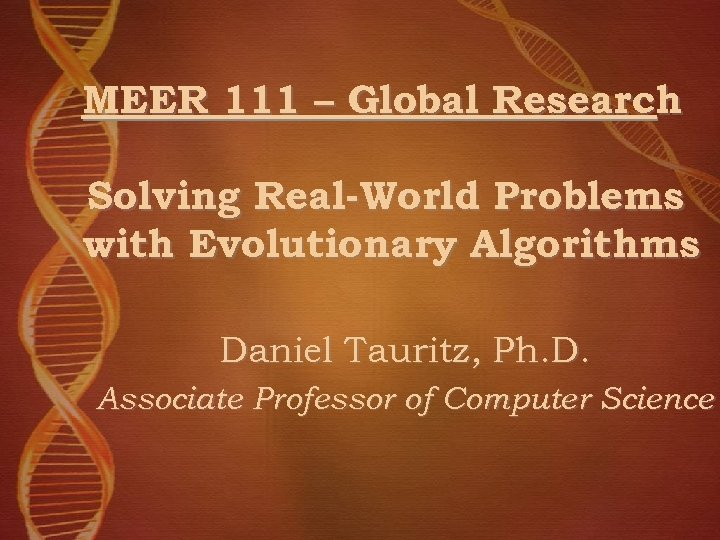MEER 111 – Global Research Solving Real-World Problems with Evolutionary Algorithms Daniel Tauritz, Ph. D. Associate Professor of Computer Science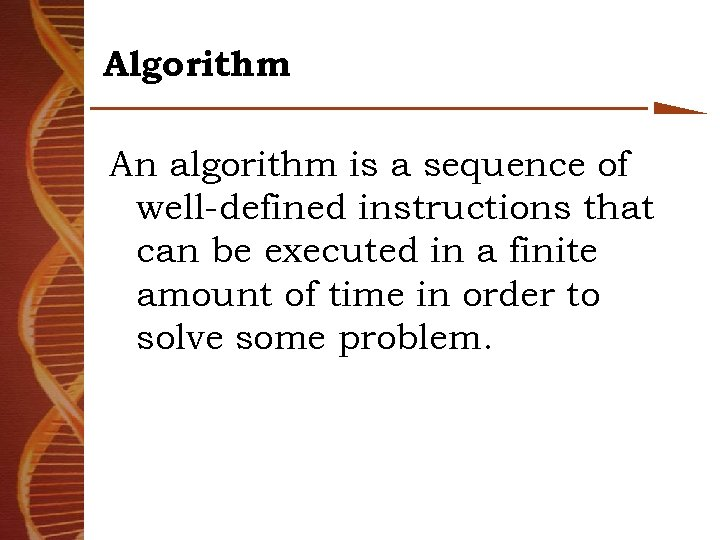Algorithm An algorithm is a sequence of well-defined instructions that can be executed in a finite amount of time in order to solve some problem.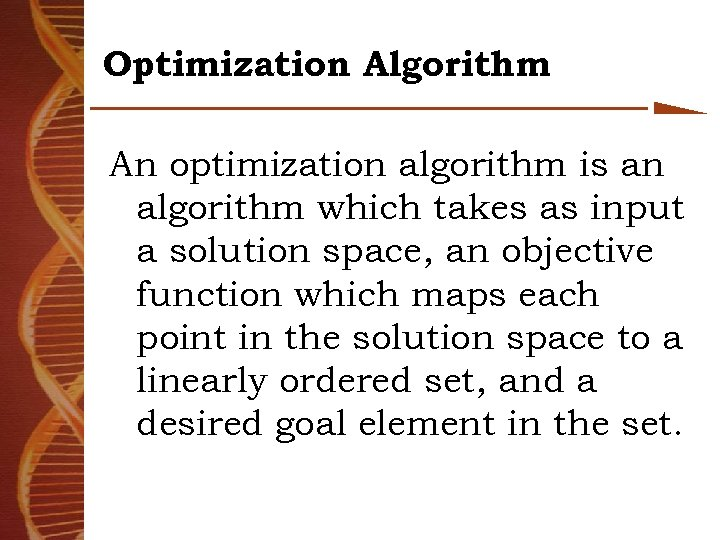Optimization Algorithm An optimization algorithm is an algorithm which takes as input a solution space, an objective function which maps each point in the solution space to a linearly ordered set, and a desired goal element in the set.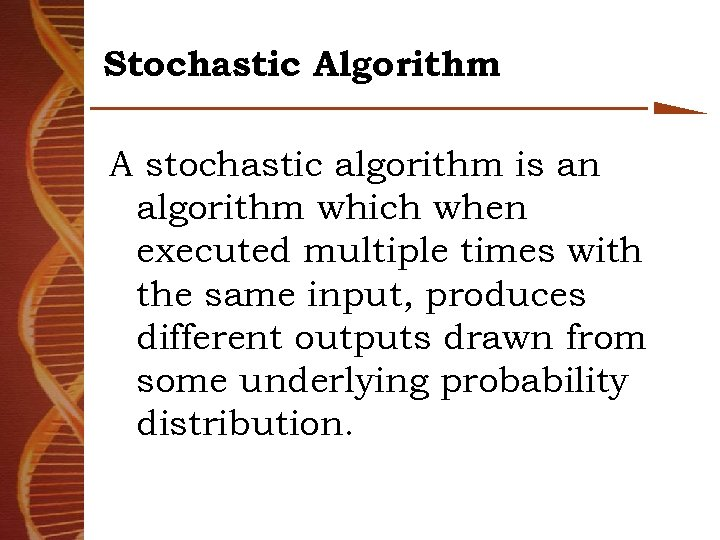Stochastic Algorithm A stochastic algorithm is an algorithm which when executed multiple times with the same input, produces different outputs drawn from some underlying probability distribution.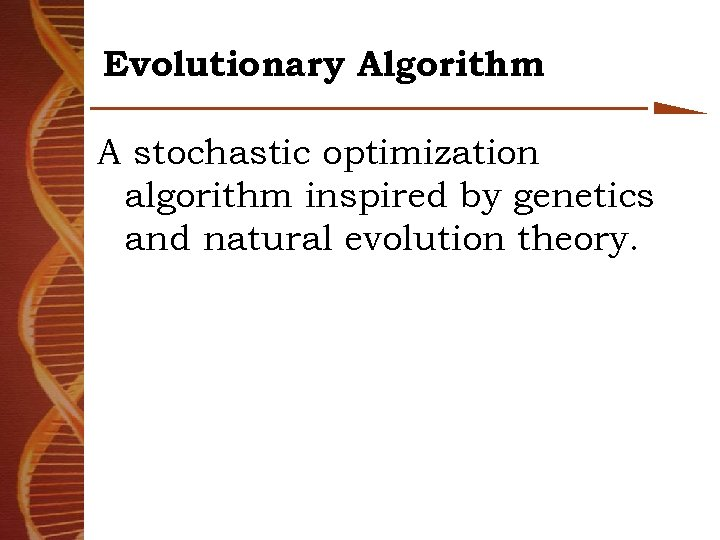Evolutionary Algorithm A stochastic optimization algorithm inspired by genetics and natural evolution theory.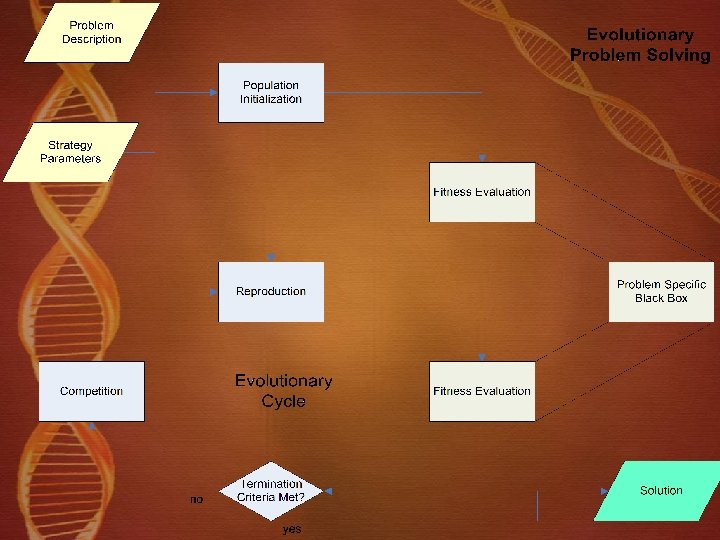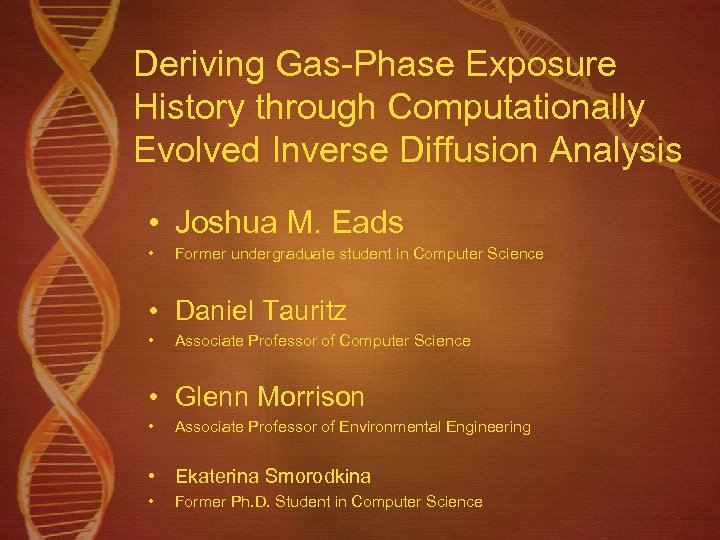Deriving Gas-Phase Exposure History through Computationally Evolved Inverse Diffusion Analysis • Joshua M. Eads • Former undergraduate student in Computer Science • Daniel Tauritz • Associate Professor of Computer Science • Glenn Morrison • Associate Professor of Environmental Engineering • Ekaterina Smorodkina • Former Ph. D. Student in Computer Science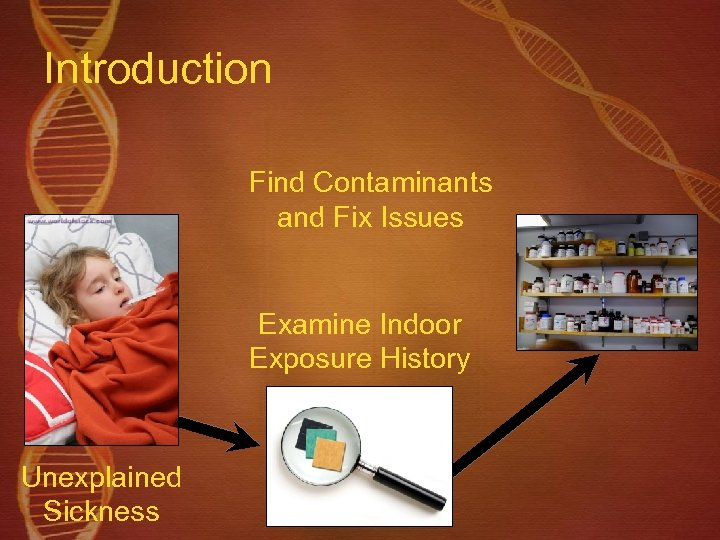Introduction Find Contaminants and Fix Issues Examine Indoor Exposure History Unexplained Sickness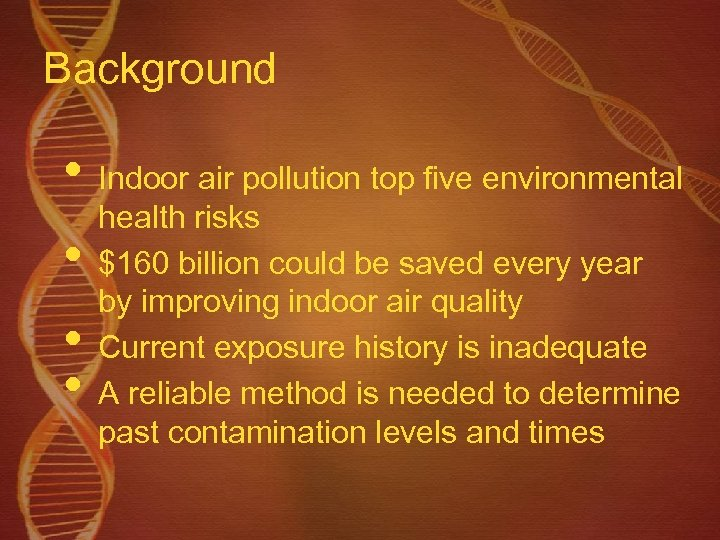Background • Indoor air pollution top five environmental • • • health risks \$160 billion could be saved every year by improving indoor air quality Current exposure history is inadequate A reliable method is needed to determine past contamination levels and times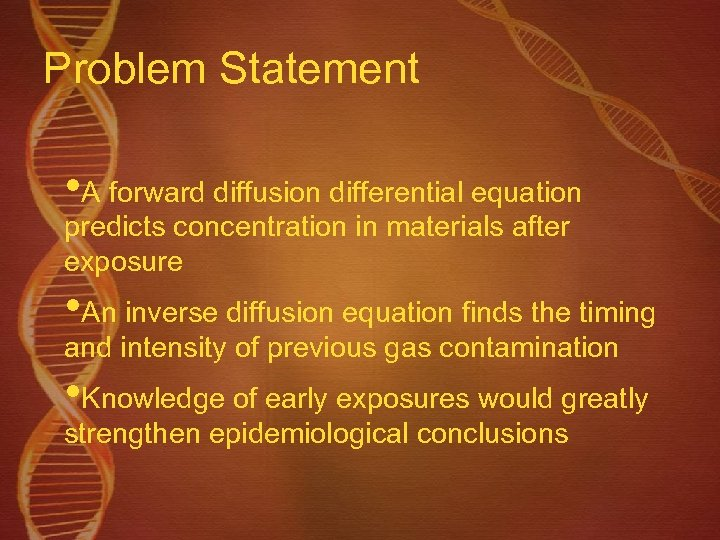Problem Statement • A forward diffusion differential equation predicts concentration in materials after exposure • An inverse diffusion equation finds the timing and intensity of previous gas contamination • Knowledge of early exposures would greatly strengthen epidemiological conclusions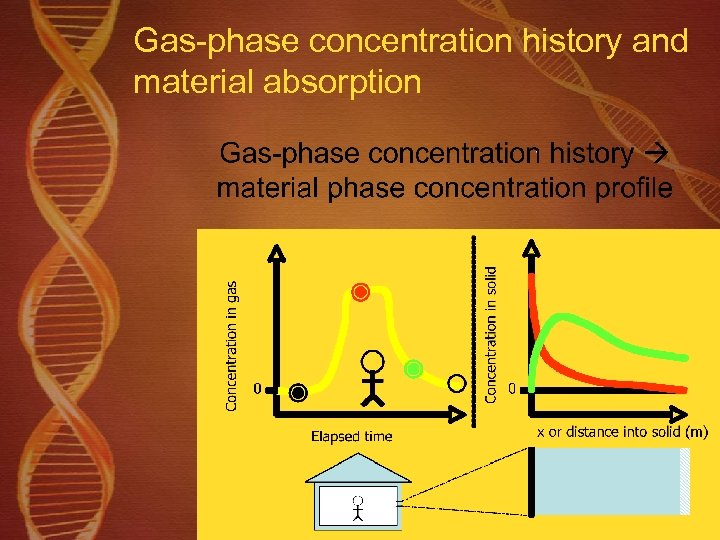Gas-phase concentration history and material absorption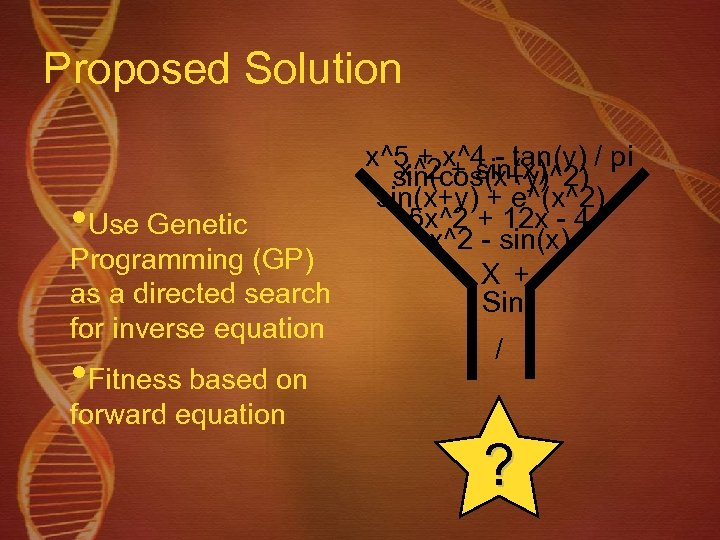Proposed Solution • Use Genetic Programming (GP) as a directed search for inverse equation • Fitness based on x^5 + x^4 - tan(y) / pi x^2 + sin(x) sin(cos(x+y)^2) sin(x+y) + e^(x^2) 5 x^2 + 12 x - 4 x^2 - sin(x) X + Sin / forward equation ?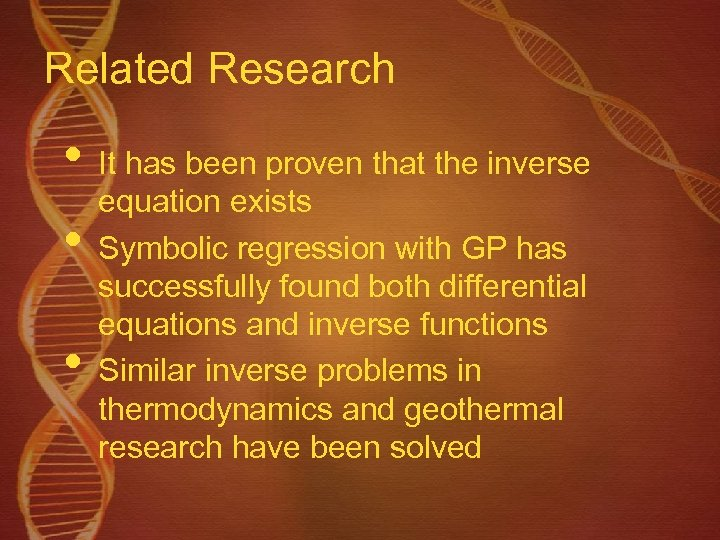Related Research • It has been proven that the inverse • • equation exists Symbolic regression with GP has successfully found both differential equations and inverse functions Similar inverse problems in thermodynamics and geothermal research have been solved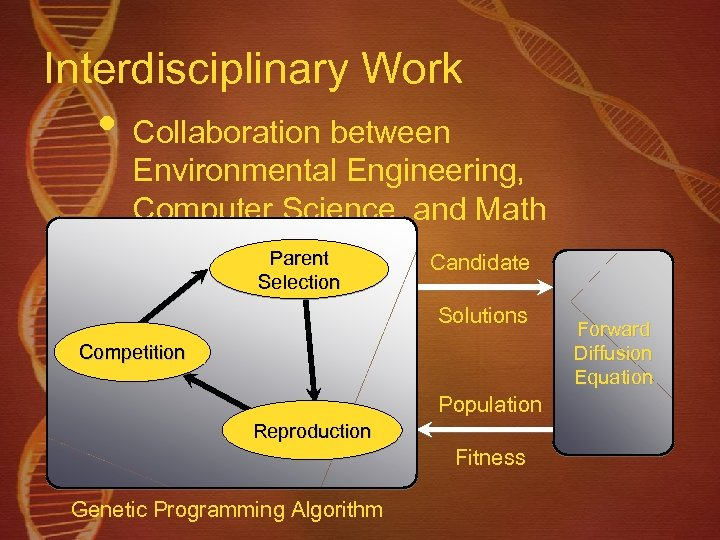Interdisciplinary Work • Collaboration between Environmental Engineering, Computer Science, and Math Parent Selection Candidate Solutions Competition Population Reproduction Fitness Genetic Programming Algorithm Forward Diffusion Equation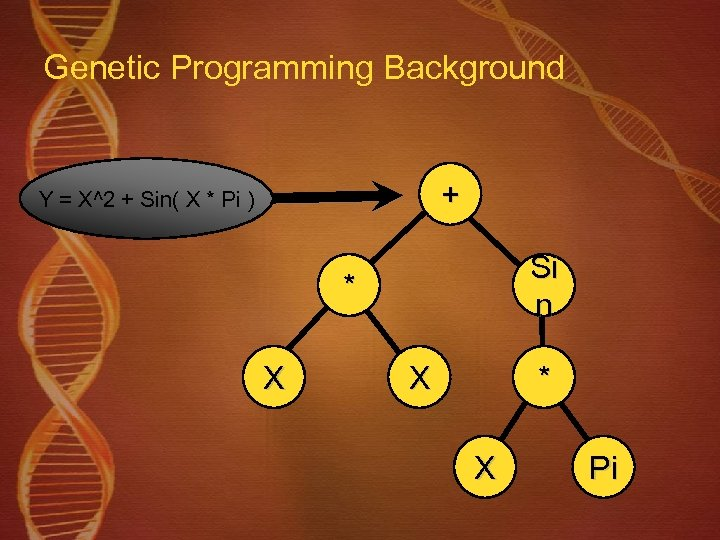Genetic Programming Background + Y = X^2 + Sin( X * Pi ) Si n * X X * X Pi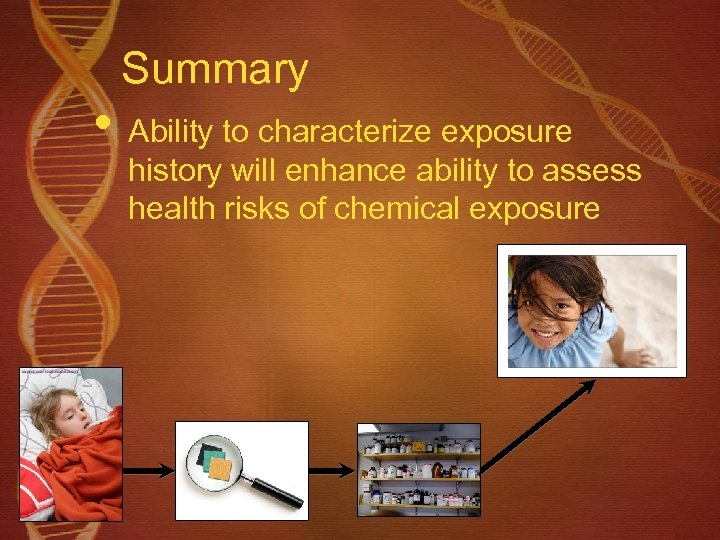Summary • Ability to characterize exposure history will enhance ability to assess health risks of chemical exposure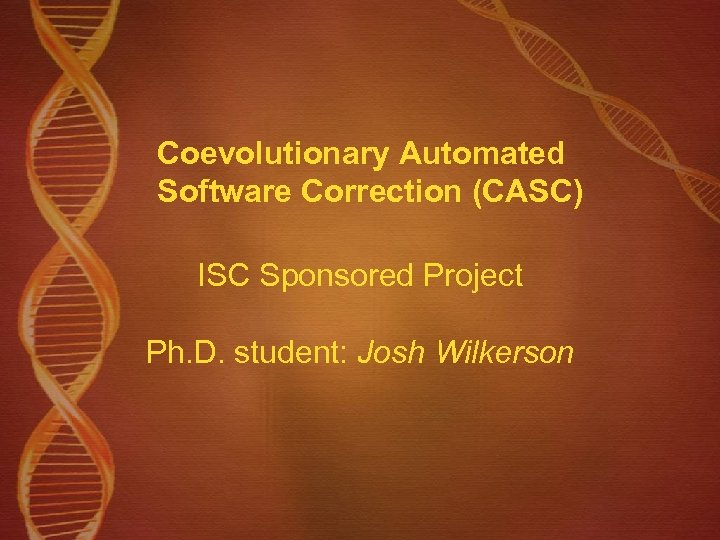Coevolutionary Automated Software Correction (CASC) ISC Sponsored Project Ph. D. student: Josh Wilkerson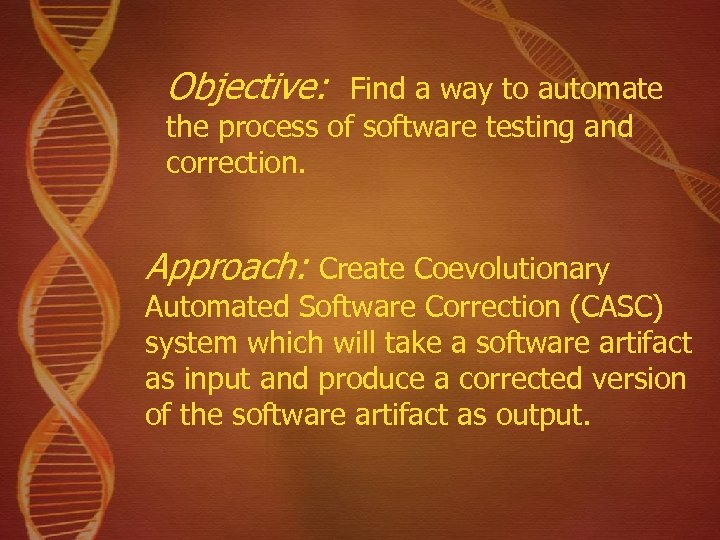Objective: Find a way to automate the process of software testing and correction. Approach: Create Coevolutionary Automated Software Correction (CASC) system which will take a software artifact as input and produce a corrected version of the software artifact as output.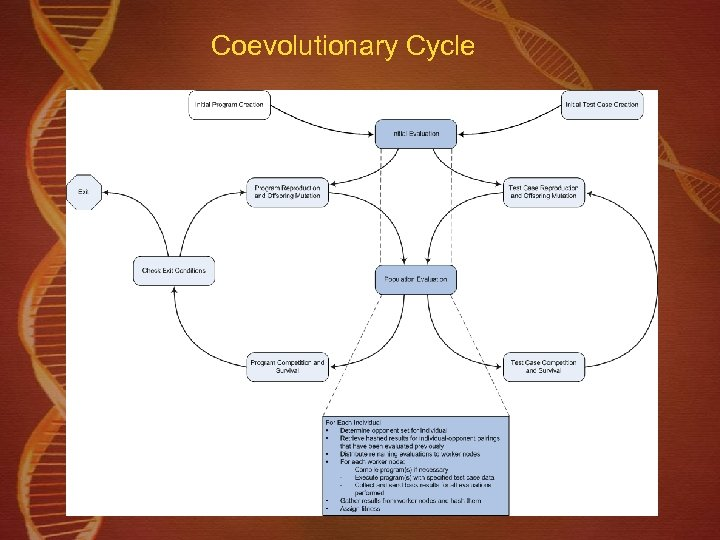Coevolutionary Cycle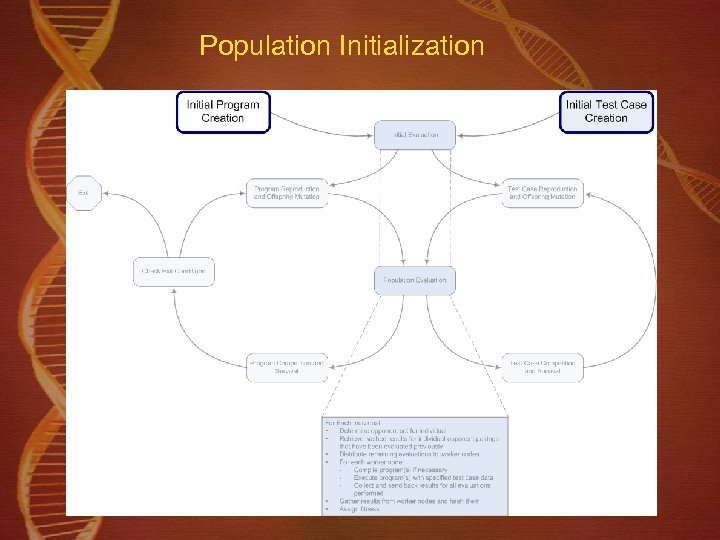Population Initialization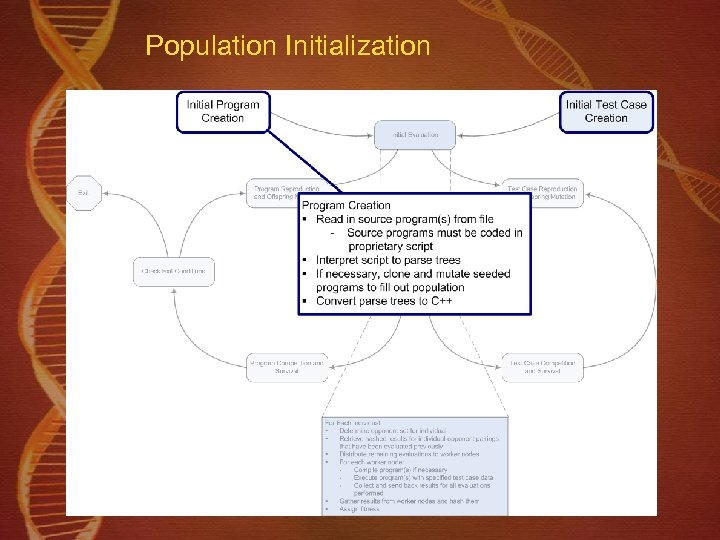Population Initialization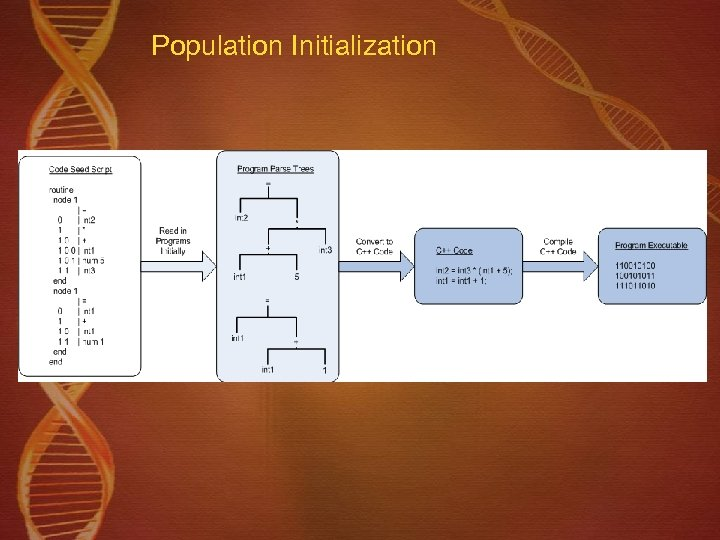Population Initialization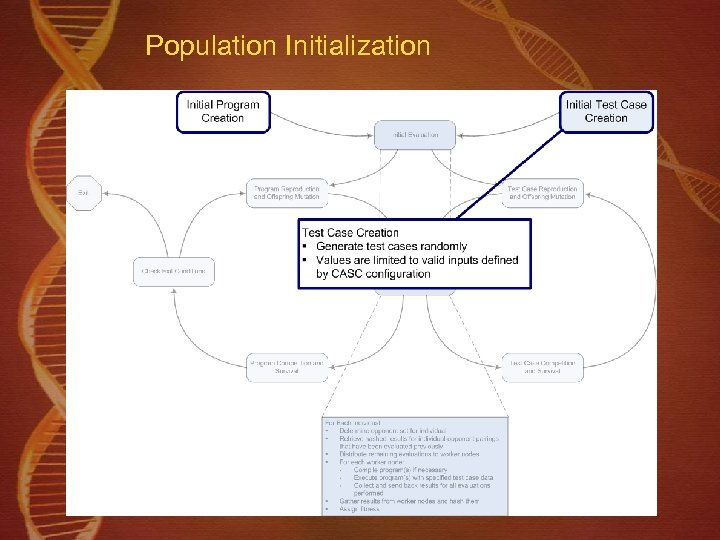Population Initialization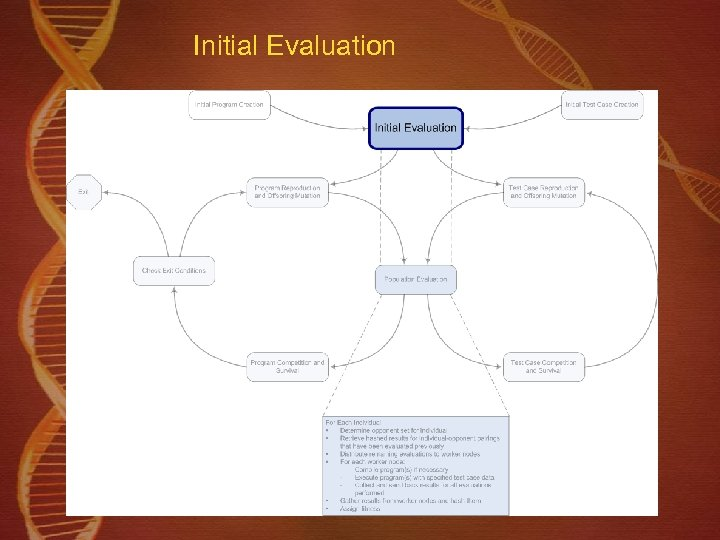Initial Evaluation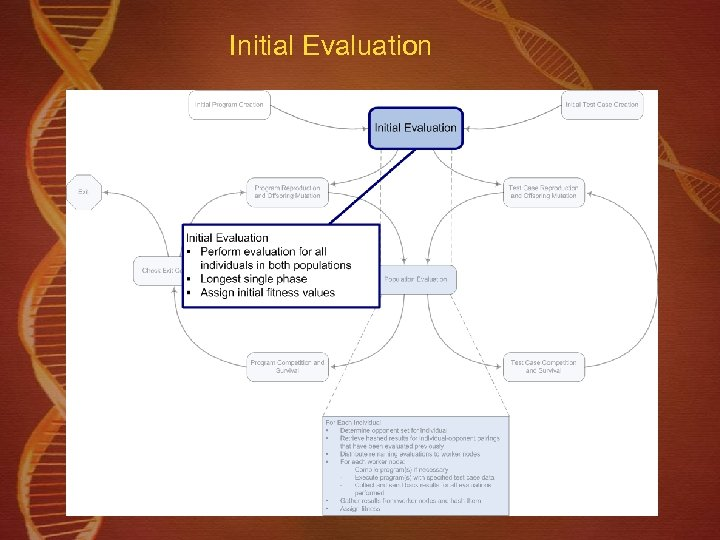Initial Evaluation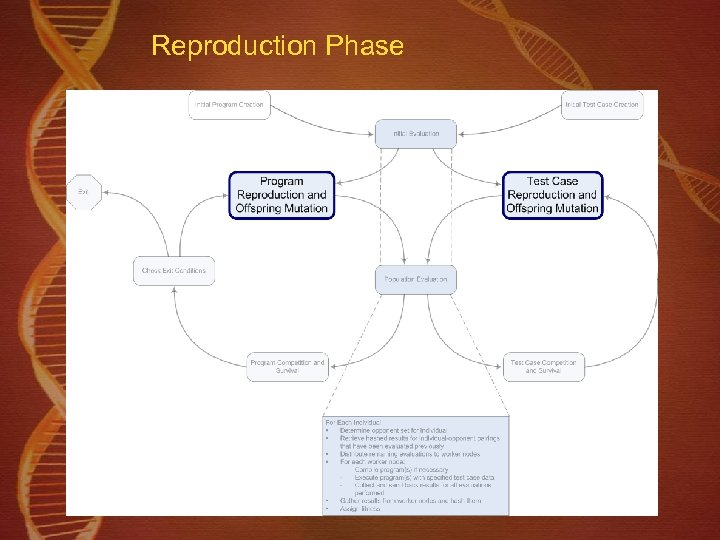Reproduction Phase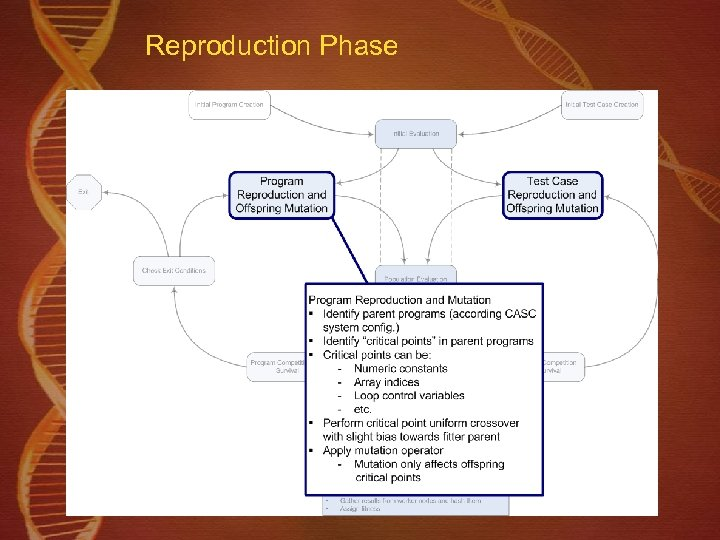Reproduction Phase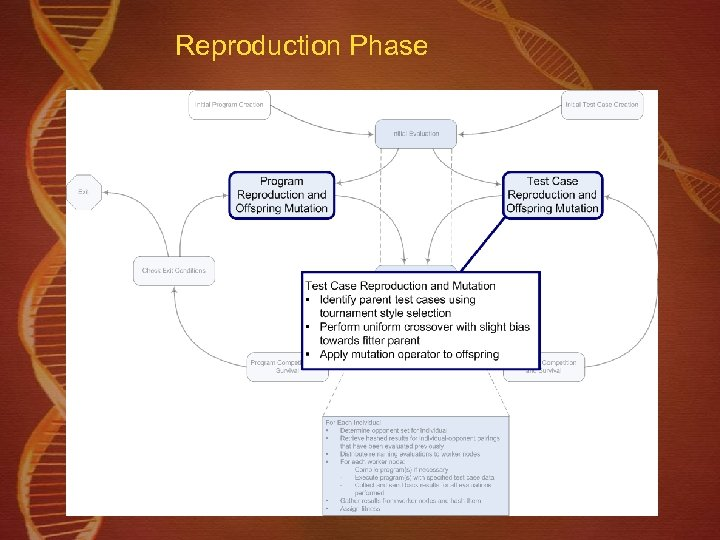Reproduction Phase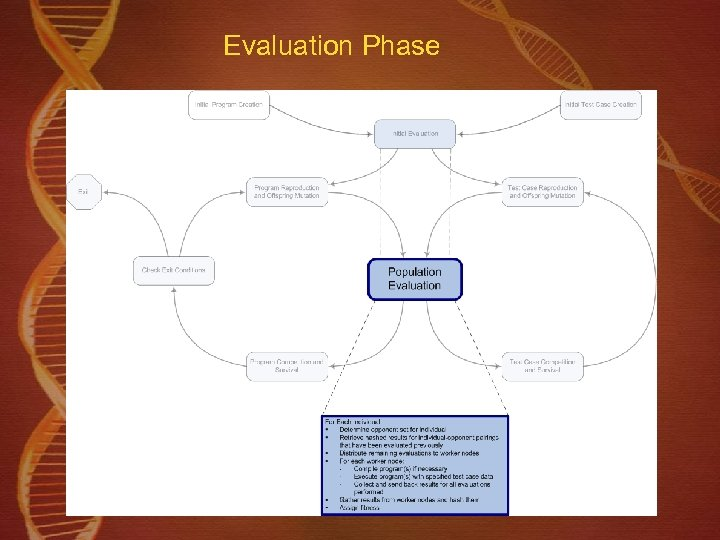Evaluation Phase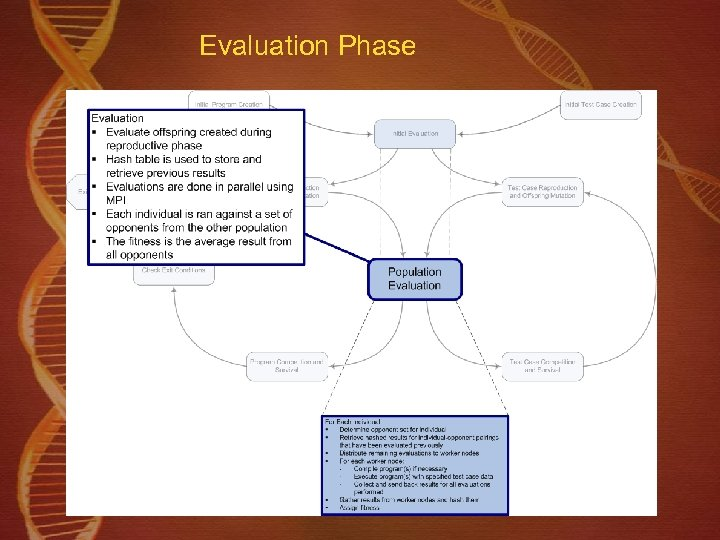Evaluation Phase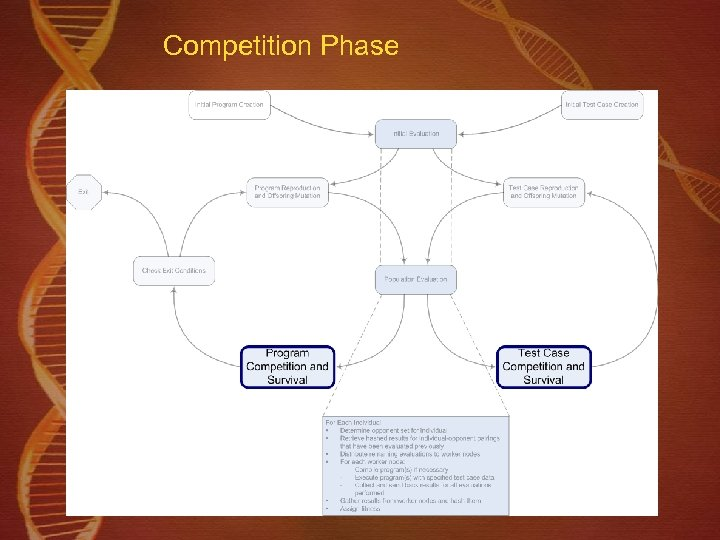Competition Phase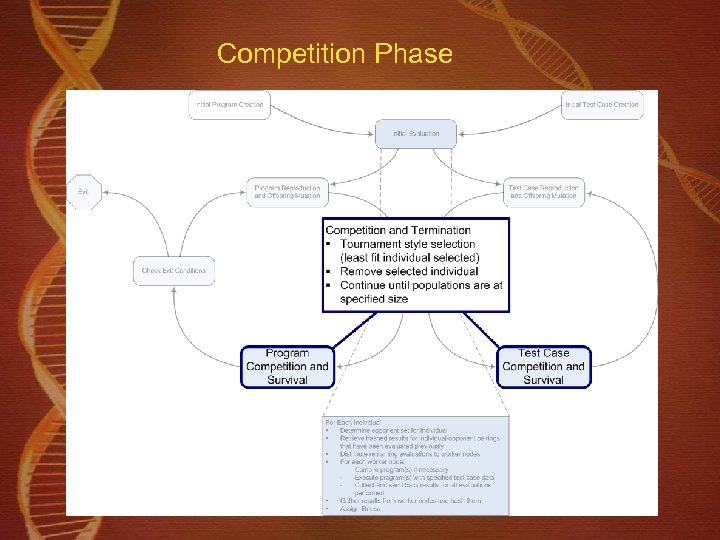Competition Phase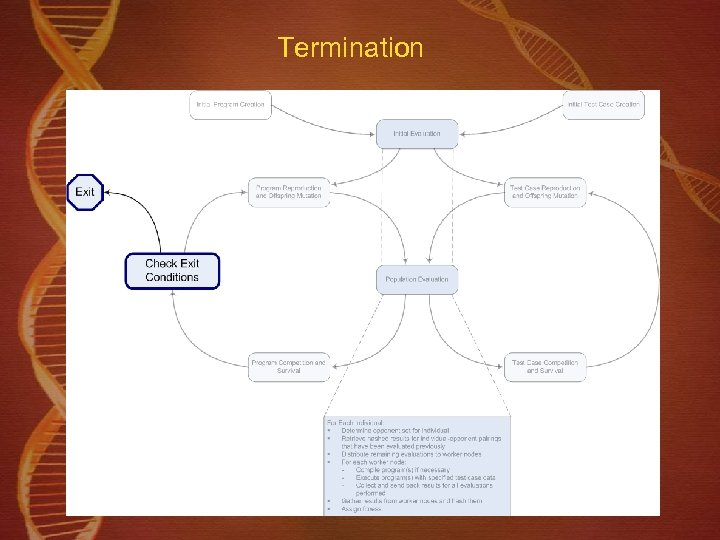Termination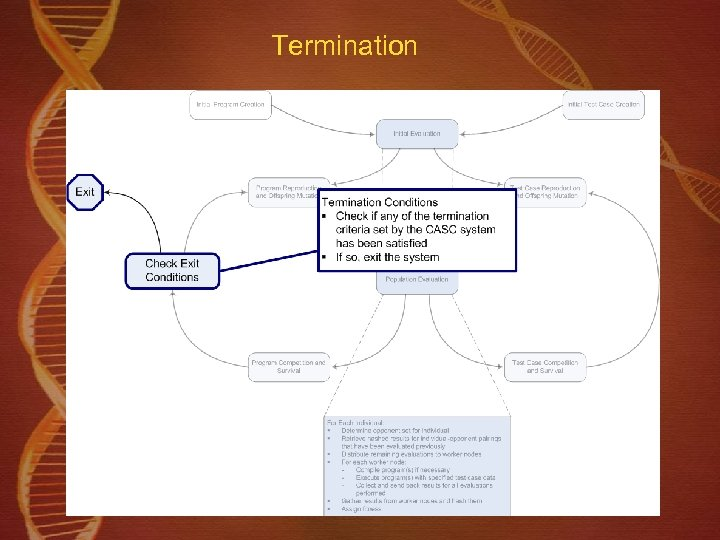Termination